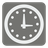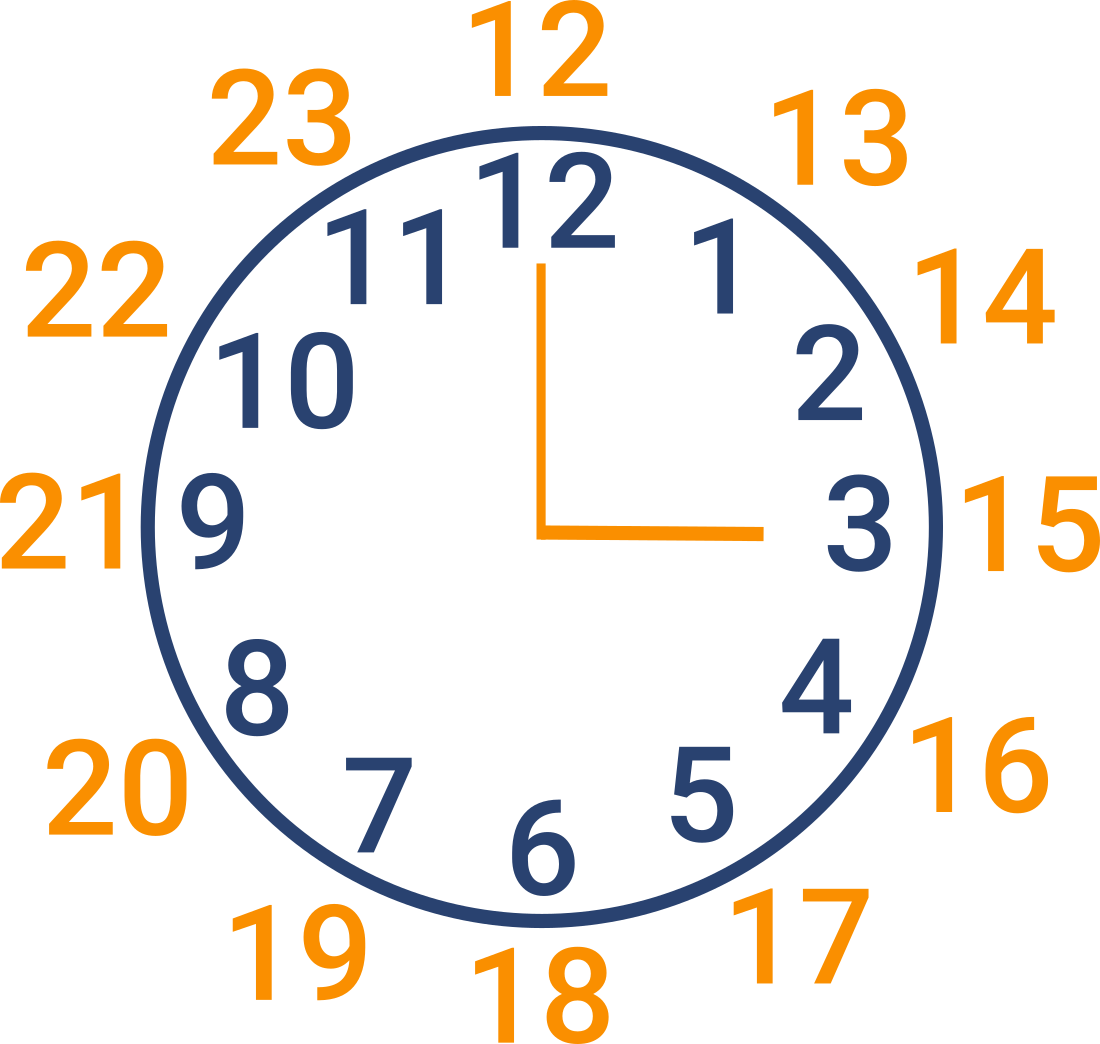Time Converter (12h to 24h)
Simple
RU English

You are the modern man who prefers the 24-hour time format. But the 12-hour format is used in some places. Your task is to convert the time from the 12-h format into 24-h by following the next rules:
- the output format should be 'hh:mm'
- if the output hour is less than 10 - write '0' before it. For example: '09:05'
Here you can find some useful information about the 12-hour format.Input: Time in a 12-hour format (as a string).

Output: Time in a 24-hour format (as a string).

Example:

```time_converter('12:30 p.m.') == '12:30'
time_converter('9:00 a.m.') == '09:00'
time_converter('11:15 p.m.') == '23:15'
```

How it is used: For work with the different time formats.

Precondition:
'00:00' <= time <= '23:59'